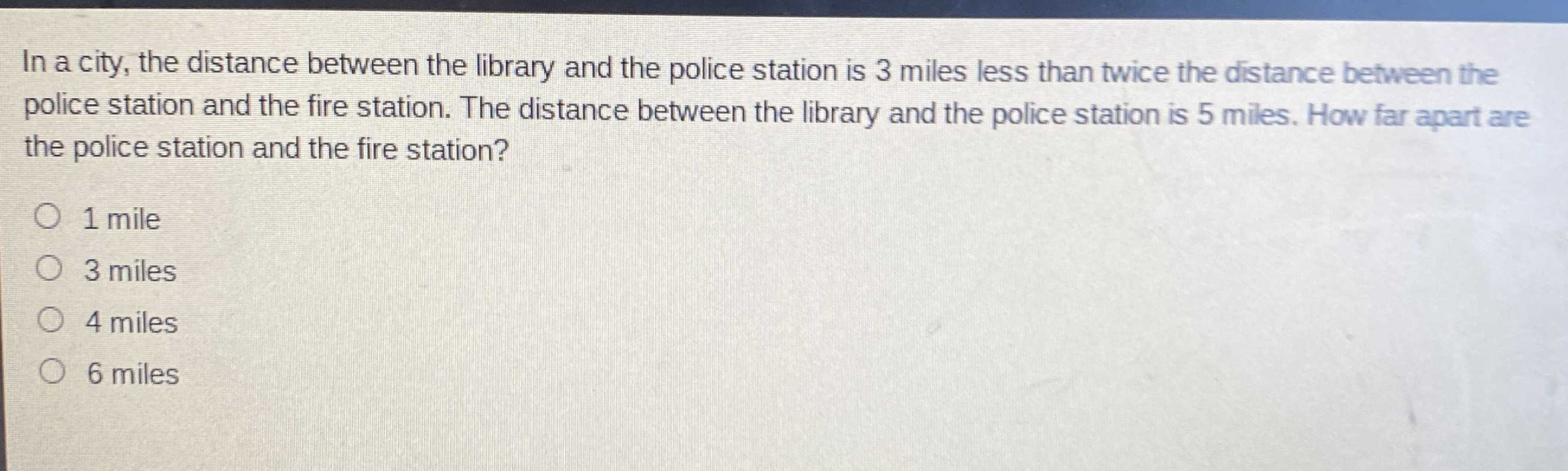### Still have math questions?

Algebra
QuestionIn a city, the distance between the library and the police station is $$3$$ miles less than twice the distance between the police station and the fire station. The distance between the library and the police station is $$5$$ miles. How far apart are the police station and the fire station?

$$\circ$$$$1$$ mile

$$\circ$$$$3$$ miles

$$\circ$$$$4$$ miles

$$\circ$$$$6$$ miles

distance between police station and library = 5

5=2x-3

2x=8

x=4

distance between police station and fire station = 4 miles

Solution
View full explanation on CameraMath App.